Updating search results...

# 28 Results

View
Selected filters:
• Reasoning and problem solving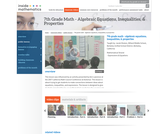Read the Fine Print
Rating
0.0 stars

This lesson is about trying to get students to make connections between ideas about equations, inequalities, and expressions. The lesson is designed to give students opportunities to use mathematical vocabulary for a purpose to describe, discuss, and work with these symbol strings.The idea is for students to start gathering global information by looking at the whole number string rather than thinking only about individual procedures or steps. Hopefully students will begin to see the symbol strings as mathematical objects with their own unique set of attributes. (7th Grade Math)

Subject:
Algebra
Numbers and Operations
Material Type:
Activity/Lab
Lecture
Lesson Plan
Teaching/Learning Strategy
Provider:
Noyce Foundation
Provider Set:
Inside Mathematics
Author:
Disston, Jacob
Date Added:
11/30/2011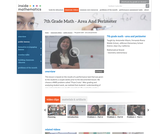Read the Fine Print
Rating
0.0 stars

This lesson is based on the results of a performance task in which we realized that students' understanding of area and perimeter was mostly procedural. Therefore the purpose of this re-engagement lesson was to address student misconceptions and deepen student understanding of area and perimeter. The standards addressed in this lesson involve finding perimeter and area of various shapes, finding the perimeter when given a fixed area, and using a formula in a practical context. Challenges for our students included decoding the language in the problem and proving their thinking. (7th Grade Math)

Subject:
Mathematics
Material Type:
Activity/Lab
Lecture
Lesson Plan
Teaching/Learning Strategy
Provider:
Noyce Foundation
Provider Set:
Inside Mathematics
Author:
Villarin, Antoinette
Date Added:
11/30/2011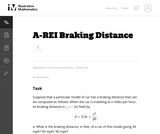Unrestricted Use
CC BY
Rating
0.0 stars

This task provides an exploration of a quadratic equation by descriptive, numerical, graphical, and algebraic techniques. Based on its real-world applicability, teachers could use the task as a way to introduce and motivate algebraic techniques like completing the square, en route to a derivation of the quadratic formula.

Subject:
Mathematics
Algebra
Functions
Material Type:
Activity/Lab
Provider:
Illustrative Mathematics
Provider Set:
Illustrative Mathematics
Author:
Illustrative Mathematics
Date Added:
05/01/2012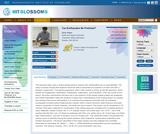Conditional Remix & Share Permitted
CC BY-NC-SA
Rating
0.0 stars

This learning video uses a simple analog setup to explore why earthquakes are so unpredictable. The setup is simple enough that students should be able to assemble and operate it on their own with a teacher's supervision. The teaching approach used in this module is known as the 5E approach, which stands for Engagement, Exploration, Explanation, Elaboration, and Evaluation. Over the course of this lesson, the basic mechanisms that give rise to the behavior of the simple analog system are explained, and further elaboration helps the students to apply their understanding of the analog system to complex fault systems that cause earthquakes

Subject:
Geology
Material Type:
Lecture
Provider:
MIT
Provider Set:
MIT Blossoms
Author:
Zach Adam
Date Added:
06/11/2012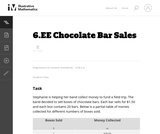Unrestricted Use
CC BY
Rating
0.0 stars

In this task students use different representations to analyze the relationship between two quantities and to solve a real world problem. The situation presented provides a good opportunity to make connections between the information provided by tables, graphs and equations.

Subject:
Mathematics
Material Type:
Activity/Lab
Provider:
Illustrative Mathematics
Provider Set:
Illustrative Mathematics
Author:
Illustrative Mathematics
Date Added:
08/08/2012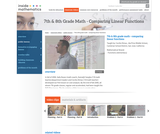Read the Fine Print
Rating
0.0 stars

The foundation of this lesson is constructing, communicating, and evaluating student-generated tables while making comparisons between three different financial plans. Students are given three different DVD rental plans and asked to analyze each one to see if they could determine when the 3 different DVD plans cost the same amount of money, if ever. (7th/8th Grade Math)

Subject:
Algebra
Material Type:
Activity/Lab
Lecture
Lesson Plan
Teaching/Learning Strategy
Provider:
Noyce Foundation
Provider Set:
Inside Mathematics
Author:
Dimas, Cecilio
Date Added:
11/30/2011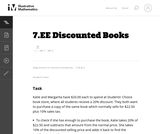Unrestricted Use
CC BY
Rating
0.0 stars

This purpose of this task is to help students see two different ways to look at percentages both as a decrease and an increase of an original amount. In addition, students have to turn a verbal description of several operations into mathematical symbols.

Subject:
Mathematics
Algebra
Material Type:
Activity/Lab
Provider:
Illustrative Mathematics
Provider Set:
Illustrative Mathematics
Author:
Illustrative Mathematics
Date Added:
05/01/2012Restricted Use
Copyright Restricted
Rating
0.0 stars

This web site offers families, teachers, and tutors 80 mathematical challenges helpful for encouraging problem solving with students in grades 6 to 8. The math challenges focus on concepts and objects found in everyday life, such as how fast your heart beats, what shape container holds the most popcorn, and how much of me shows in a mirror. Each challenge contains an initial problem with a solution hint, a complete explanation of the answer, and additional problems related to the same challenge. Resources for further investigations are suggested as well. From the Printing the Challenges link on the homepage, PDF files are available for all 80 challenges in English, the first 15 challenges in Spanish, and the family resource materials in English and Spanish.

Subject:
Algebra
Geometry
Statistics and Probability
Material Type:
Activity/Lab
Homework/Assignment
Provider:
National Council of Teachers of Mathematics
Provider Set:
Figure This!
Date Added:
01/01/2002Unrestricted Use
CC BY
Rating
0.0 stars

In this task students are asked to write an equation to solve a real-world problem. There are two natural approaches to this task. In the first approach, students have to notice that even though there is one variable, namely the number of firefighters, it is used in two different places. In the other approach, students can find the total cost per firefighter and then write the equation.

Subject:
Mathematics
Material Type:
Activity/Lab
Provider:
Illustrative Mathematics
Provider Set:
Illustrative Mathematics
Author:
Illustrative Mathematics
Date Added:
05/01/2012Conditional Remix & Share Permitted
CC BY-NC-SA
Rating
0.0 stars

This video lesson shows students that math can play a role in understanding how an infectious disease spreads and how it can be controlled. During this lesson, students will see and use both deterministic and probabilistic models and will learn by doing through role-playing exercises. The primary exercises between video segments of this lesson are class-intensive simulation games in which members of the class 'infect' each other under alternative math modeling assumptions about disease progression. Also there is an occasional class discussion and local discussion with nearby classmates.

Subject:
Biology
Sociology
Material Type:
Lecture
Provider:
MIT
Provider Set:
MIT Blossoms
Author:
Mai Perches
Richard C. Larson
Sahar Hashmi
Date Added:
07/12/2014Conditional Remix & Share Permitted
CC BY-NC-SA
Rating
0.0 stars

This video lesson shows students that math can play a role in understanding how an infectious disease spreads and how it can be controlled. During this lesson, students will see and use both deterministic and probabilistic models and will learn by doing through role-playing exercises. The primary exercises between video segments of this lesson are class-intensive simulation games in which members of the class 'infect' each other under alternative math modeling assumptions about disease progression. Also there is an occasional class discussion and local discussion with nearby classmates.

Subject:
Biology
Sociology
Material Type:
Lecture
Provider:
MIT Learning International Networks Consortium
Provider Set:
M.I.T. Blossoms
Author:
Mai Perches
Richard C. Larson
Sahar Hashmi
Date Added:
06/02/2012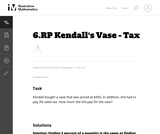Unrestricted Use
CC BY
Rating
0.0 stars

This word problem requires students to compute sales tax.

Subject:
Mathematics
Material Type:
Activity/Lab
Provider:
Illustrative Mathematics
Provider Set:
Illustrative Mathematics
Author:
Illustrative Mathematics
Date Added:
05/01/2012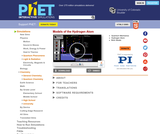Unrestricted Use
CC BY
Rating
0.0 stars

How did scientists figure out the structure of atoms without looking at them? Try out different models by shooting light at the atom. Check how the prediction of the model matches the experimental results.

Subject:
Physics
Material Type:
Simulation
Provider:
University of Colorado Boulder
Provider Set:
PhET Interactive Simulations
Author:
Carl Wieman
Chris Malley
Kathy Perkins
Michael Dubson
Mindy Gratny
Sam McKagan
Wendy Adams
Date Added:
01/01/2007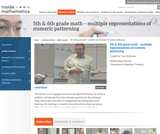Read the Fine Print
Rating
3.0 stars

This lesson is a re-engagement lesson designed for learners to revisit a problem-solving task they have already experienced. Students will activate prior knowledge of graphical representations through the 'what's my rule' number talk; compare and contrast two different learners' interpretations of the growing pattern; use multiple representations to demonstrate how one of these learners would represent the numeric pattern; make connections between the different representations to more critically compare the two interpretations. (5th/6th Grade Math)

Subject:
Numbers and Operations
Material Type:
Activity/Lab
Lecture
Lesson Plan
Teaching/Learning Strategy
Provider:
Noyce Foundation
Provider Set:
Inside Mathematics
Author:
Dickinson, Fran
Date Added:
11/30/2011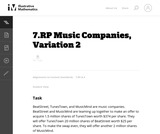Unrestricted Use
CC BY
Rating
0.0 stars

This real world word problem requires multiple steps.

Subject:
Mathematics
Material Type:
Activity/Lab
Provider:
Illustrative Mathematics
Provider Set:
Illustrative Mathematics
Author:
Illustrative Mathematics
Date Added:
05/01/2012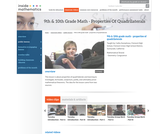Read the Fine Print
Rating
0.0 stars

This lesson is about properties of quadrilaterals and learning to investigate, formulate, conjecture, justify, and ultimately prove mathematical theorems. Students will: Analyze characteristics and properties of two- and three-dimensional geometric shapes; develop mathematical arguments about geometric relationships; and apply appropriate techniques, tools, and formulas to determine measurements.Explore relationships among classes of two- and three-dimensional geometric objects, make and test conjectures about them, and solve problems involving them. Employ forms of mathematical reasoning and proof appropriate to the solution of the problem at hand, including deductive and inductive reasoning, making and testing conjectures, and using counter examples and indirect proof. Identify, formulate and confirm conjectures. Establish the validity of geometric conjectures using deduction, prove theorems, and critique arguments made by others. (9th/10th Grade Math)

Subject:
Algebra
Material Type:
Activity/Lab
Lecture
Lesson Plan
Teaching/Learning Strategy
Provider:
Noyce Foundation
Provider Set:
Inside Mathematics
Author:
Humphreys, Cathy
Date Added:
11/30/2011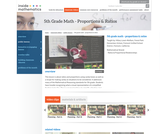Read the Fine Print
Rating
0.0 stars

This lesson is about ratios and proportions using candy boxes as well as a recipe for making candy as situations to be considered. It addresses many Mathematical Reasoning standards and asks students to: Use models to understand fractions and to solve ratio problems; think about a ratio as part/part model and to think about the pattern growing in equal groups or a unit composed of the sum of the parts; find a scale factor and apply it to a ratio. (5th Grade Math)

Subject:
Ratios and Proportions
Material Type:
Activity/Lab
Lecture
Lesson Plan
Teaching/Learning Strategy
Provider:
Noyce Foundation
Provider Set:
Inside Mathematics
Author:
Lewis-Wolfsen, Hillary
Date Added:
11/30/2011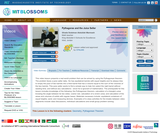Conditional Remix & Share Permitted
CC BY-NC-SA
Rating
0.0 stars

This video lesson presents a real world problem that can be solved by using the Pythagorean theorem. The problem faces a juice seller daily. He has equilateral barrels with equal heights and he always tries to empty the juice of two barrels into a third barrel that has a volume equal to the sum of the volumes of the two barrels. This juice seller wants to find a simple way to help him select the right barrel without wasting time, and without any calculations - since he is ignorant of Mathematics. The prerequisite for this lesson includes knowledge of the following: the Pythagorean theorem; calculation of a triangles area knowing the angle between its two sides; cosine rule; calculation of a circle's area; and calculation of the areas and volumes of solids with regular bases.

Subject:
Geometry
Measurement and Data
Material Type:
Lecture
Provider:
MIT
Provider Set:
MIT Blossoms
Author:
Ghada Sulaiman Abdullah Marmash
Date Added:
06/02/2012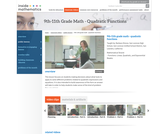Read the Fine Print
Rating
0.0 stars

This lesson focuses on students making decisions about what tools to apply to solve different problems related to quadratic expressions and equations. It is also intended to build awareness of the form an answer will take in order to help students make sense of the kind of problem they are solving. (9th/10th/11th Grade Math)

Subject:
Algebra
Material Type:
Activity/Lab
Lecture
Lesson Plan
Teaching/Learning Strategy
Provider:
Noyce Foundation
Provider Set:
Inside Mathematics
Author:
Shreve, Barbara
Date Added:
11/30/2011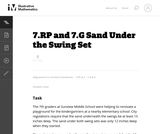Unrestricted Use
CC BY
Rating
0.0 stars

This real world word problem requires students to figure out the scale ratio and unit rate.

Subject:
Mathematics
Geometry
Material Type:
Activity/Lab
Provider:
Illustrative Mathematics
Provider Set:
Illustrative Mathematics
Author:
Illustrative Mathematics
Date Added:
05/01/2012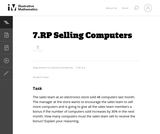Unrestricted Use
CC BY
Rating
0.0 stars

There are a couple of ways to solve this real world word problem.

Subject:
Mathematics
Material Type:
Activity/Lab
Provider:
Illustrative Mathematics
Provider Set:
Illustrative Mathematics
Author:
Illustrative Mathematics
Date Added:
05/01/2012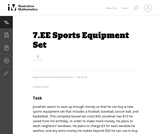Unrestricted Use
CC BY
Rating
0.0 stars

The purpose of this task is to present students with a context that can naturally be represented with an inequality and to explore the relationship between the context and the mathematical representation of that context; thus, this is an intended as an instructional task.

Subject:
Mathematics
Material Type:
Activity/Lab
Provider:
Illustrative Mathematics
Provider Set:
Illustrative Mathematics
Author:
Illustrative Mathematics
Date Added:
08/10/2012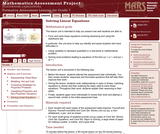Only Sharing Permitted
CC BY-NC-ND
Rating
5.0 stars

This lesson unit is intended to help teachers assess how well students are able to: form and solve linear equations involving factorizing and using the distributive law. In particular, this unit aims to help teachers identify and assist students who have difficulties in: using variables to represent quantities in a real-world or mathematical problem and solving word problems leading to equations of the form px + q = r and p(x + q) = r.

Subject:
Algebra
Material Type:
Assessment
Lesson Plan
Provider:
Shell Center for Mathematical Education
Provider Set:
Mathematics Assessment Project (MAP)
Date Added:
04/26/2013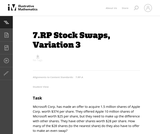Unrestricted Use
CC BY
Rating
0.0 stars

This is a multi-step problem since it requires more than two steps no matter how it is solved. The problem is not scaffolded for the student, but each step is straightforward and should follow from the previous with a careful reading of the problem.

Subject:
Mathematics
Material Type:
Activity/Lab
Provider:
Illustrative Mathematics
Provider Set:
Illustrative Mathematics
Author:
Illustrative Mathematics
Date Added:
05/01/2012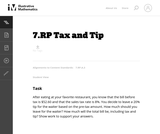Unrestricted Use
CC BY
Rating
0.0 stars

This real world word problem about calculating a tip in a restaurant requires multiple steps.

Subject:
Mathematics
Material Type:
Activity/Lab
Provider:
Illustrative Mathematics
Provider Set:
Illustrative Mathematics
Author:
Illustrative Mathematics
Date Added:
05/01/2012Unrestricted Use
CC BY
Rating
0.0 stars

This real world word problem requires students to use equations and functions.

Subject:
Mathematics
Functions
Material Type:
Activity/Lab
Provider:
Illustrative Mathematics
Provider Set:
Illustrative Mathematics
Author:
Illustrative Mathematics
Date Added:
05/01/2012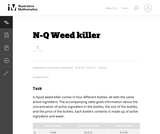Unrestricted Use
CC BY
Rating
0.0 stars

The principal purpose of the task is to explore a real-world application problem with algebra, working with units and maintaining reasonable levels of accuracy throughout.

Subject:
Mathematics
Algebra
Material Type:
Activity/Lab
Provider:
Illustrative Mathematics
Provider Set:
Illustrative Mathematics
Author:
Illustrative Mathematics
Date Added:
05/01/2012Unrestricted Use
CC BY
Rating
0.0 stars

In this real world word problem students must graph linear equations.

Subject:
Mathematics
Material Type:
Activity/Lab
Provider:
Illustrative Mathematics
Provider Set:
Illustrative Mathematics
Author:
Illustrative Mathematics
Date Added:
05/01/2012